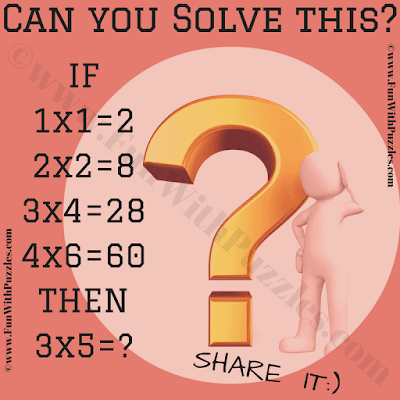This is an Easy Logical Reasoning Question of Mathematics for school going Kids. This Logical Reasoning Question will test your Mathematical Skills and Logical Reasoning Ability. In  this Brain Teaser your challenge is to decipher the Number Equations and find the value of the missing number which will replace the question mark. Lets see if you can solve this Logical Reasoning Question of Mathematics?Can you solve this Logical Reasoning Question?